Smartick is a fun way to learn math!Jul28

# Equivalent Fractions

### What are equivalent fractions?

In this post, we are going to understand what equivalent fractions are and how you can find out what fractions are equivalent.

Equivalent fractions are fractions that represent the same quantity. For example, which of the following do you believe will be the biggest?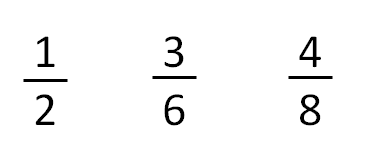What have you figured out? We are going to see it with an example, sharing this pizza in as many slices as the fraction indicates.In order to represent 1/2, we will divide the pizza into 2 slices and keep 1 slice for ourselves: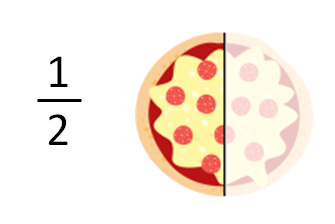In order to represent 3/6, we will divide the pizza into 6 slices and keep 3 slices for ourselves: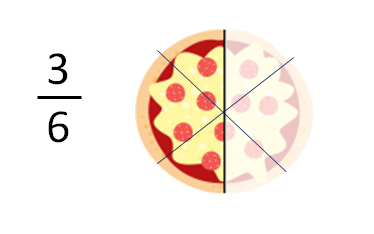In order to represent 4/8, we will divide the pizza into 8 slices and keep 4 slices for ourselves: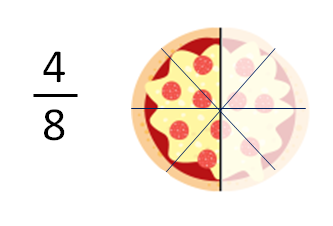Is there a piece of pizza that is bigger? No! Notice, the three fractions represent the same quantity of pizza, one half; therefore those are equivalent fractions: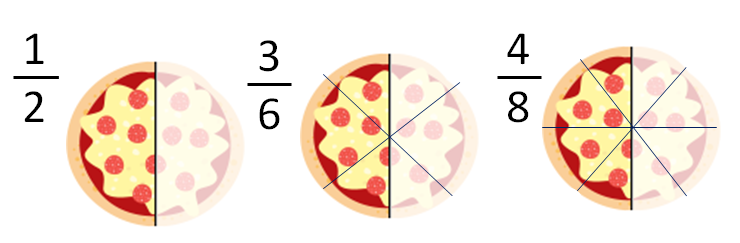### How do we know if two fractions are equivalent?

Two fractions are equivalent if they represent the same decimal number.

For example, the three previous fractions represent the same decimal: 0.5.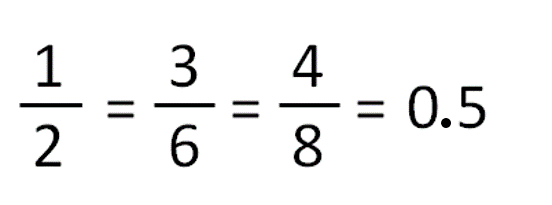1/2 is 1 between 2, which is 0.5.

3/6 is 3 between 6, which is 0.5.

4/8 is 4 entre 8, which is 0.5.

### How are we able to find a fraction equivalent to another?

If we want to find an equivalent fraction to another, we can:

• Multiply the denominator and the numerator by the same number. We will get an equivalent fraction with bigger numbers. This process is called magnification.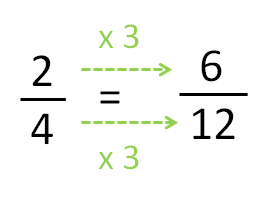• Divide the denominator and numerator by the same number (both must be divisible by the same number). This way, we get an equivalent fraction with smaller numbers. This process is called simplification.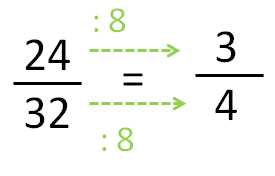Are you ready to practice some exercises with equivalent fractions? Try out the following links:

Fun is our brain’s favorite way of learning
Diane Ackerman
Smartick is a fun way to learn math
• 15 fun minutes a day
• Millions of students since 2009